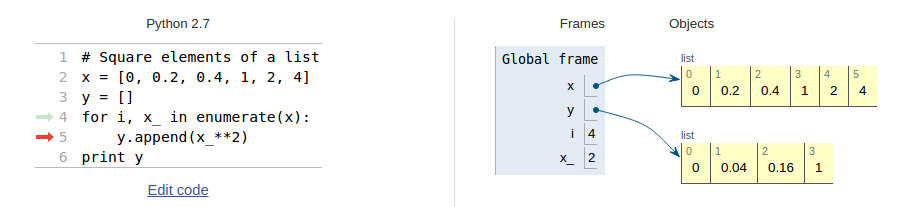$$\newcommand{\Oof}{\mathcal{O}(#1)} \newcommand{\F}{\boldsymbol{F}} \newcommand{\J}{\boldsymbol{J}} \newcommand{\x}{\boldsymbol{x}} \renewcommand{\c}{\boldsymbol{c}}$$

# Basic constructions# If tests, colon and indentation

Very often in life, and in computer programs, the next action depends on the outcome of a question starting with "if". This gives the possibility to branch into different types of action depending on some criterion. Let us as usual focus on a specific example, which is the core of so-called random walk algorithms used in a wide range of branches in science and engineering, including materials manufacturing and brain research. The action is to move randomly to the north (N), east (E), south (S), or west (W) with the same probability. How can we implement such an action in life and in a computer program?

We need to randomly draw one out of four numbers to select the direction in which to move. A deck of cards can be used in practice for this purpose. Let the four suits correspond to the four directions: clubs to N, diamonds to E, hearts to S, and spades to W, for instance. We draw a card, perform the corresponding move, and repeat the process a large number of times. The resulting path is a typical realization of the path of a diffusing molecule.

In a computer program, we need to draw a random number, and depending on the number, update the coordinates of the point to be moved. There are many ways to draw random numbers and translate them into (e.g.) four random directions, but the technical details usually depend on the programming language. Our technique here is universal: we draw a random number in the interval $$[0,1)$$ and let $$[0,0.25)$$ correspond to N, $$[0.25,0.5)$$ to E, $$[0.5,0.75)$$ to S, and $$[0.75,1)$$ to W. Let x and y hold the coordinates of a point and let d be the length of the move. A pseudo code (i.e., not "real" code, just a "sketch of the logic") then goes like

r = random number in [0,1)
if 0 <= r < 0.25
move north: y = y + d
else if 0.25 <= r < 0.5
move east:  x = x + d
else if 0.5 <= r < 0.75
move south:  y = y - d
else if 0.75 <= r < 1
move west:  x = x - d


Note the need for first asking about the value of r and then performing an action. If the answer to the "if" question is positive (true), we are done and can skip the next else if questions. If the answer is negative (false), we proceed with the next question. The last test if $$0.75\leq r < 1$$ could also read just else, since we here cover all the remaining possible r values.

The exact code in Python reads

import random
r = random.random()        # random number in [0,1)
if 0 <= r < 0.25:
# move north
y = y + d
elif 0.25 <= r < 0.5:
# move east
x = x + d
elif 0.5 <= r < 0.75:
# move south
y = y - d
else:
# move west
x = x - d


We use else in the last test to cover the different types of syntax that is allowed. Python recognizes the reserved words if, elif (short for else if), and else and expects the code to be compatible with the rules of if tests:

• The test reads if condition:, elif condition:, or else:, where condition is a boolean expression that evaluates to True or False. Note the closing colon (easy to forget!).
• If condition is True, the following indented block of statements are executed and the remaining if, elif, or else branches are skipped.
• If condition is False, the program flow jumps to the next if, elif, or else branch.
The blocks after if, elif, or else may contain new if tests, if desired. Regarding colon and indent, you will see below that these are required in several other programming constructions as well.

Working with if tests requires mastering boolean expressions. Here are some basic boolean expressions involving the logical operators ==, !=, <, <=, >, and >=. Given the assignment to temp, you should go through each boolean expression below and determine if it is true or false.

temp = 21       # assign value to a variable
temp == 20	# temp equal to 20
temp != 20	# temp not equal to 20
temp <  20	# temp less than 20
temp >  20	# temp greater than 20
temp <= 20	# temp less than or equal to 20
temp >= 20	# temp greater than or equal to 20